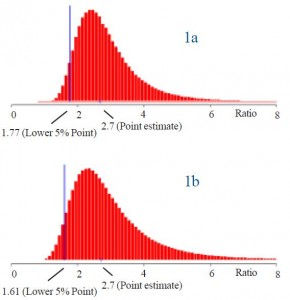## TECHNICAL REPORTS

Making Ratio Statements about Product Improvements

Ennis, D. M. (2006). IFPress, 9(2) 2-3.

Abstract:

When making product comparisons, it is sometimes useful to be able to substantiate ratio statements. The purpose of this report is to consider how this might be accomplished using standard rating and difference testing methods.

In everyday experience, such as finding out what the time or temperature is, we often use interval scales. When we say that it is 8:30am, we mean that an interval of eight hours and thirty minutes has elapsed since midnight, which is an arbitrarily agreed-upon point to begin recording time for the new day. This is our zero point on an interval scale on which the difference between each number has the same meaning. The time elapsed between 8am and 7am is the same as that between 7am and 6am. We use the same ideas when we use Centigrade (Celsius) and Fahrenheit scales to record temperature. If we take ratios of the numbers on these scales, the results are not meaningful. For instance, although 100°C/20°C is a ratio of 5, the corresponding ratio for the same temperatures in Fahrenheit is 212°F/68°F which is not 5, it is 3.1. The same difficulty arises with time as we usually measure it. We could measure temperature and time on absolute scales for which there is an absolute zero point, such as the Kelvin scale, but for time we would have to measure time from the beginning of the universe. If this is really the case, how can we make ratio statements such as: “It took me twice as long to get to work today as it did yesterday”? The answer is that, although interval scales do not have ratio properties, distances between points on them do. For interval scales linearly related to each other, the ratios are the same for equivalent points on the scales. Using the Centigrade scale |20 - 0 |/|100 - 0| = 1/5; and using the same temperatures in Fahrenheit |68 - 32|/|212 - 32| = 1/5. Similarly, the statement about getting to work is a ratio of distances on the interval time scale and we don’t need to know how old the universe is to make it.Figure 1. Distributions of ratios of malodor improvement for rating (1a) and 2-AFC experiments (1b). In both cases, a point estimate of the ratio is 2.7. For ratings the lower 5% point for the ratio is 1.77 and is 1.61 for the 2-AFC